The resultant capacitance between A and B in the following figure is equal to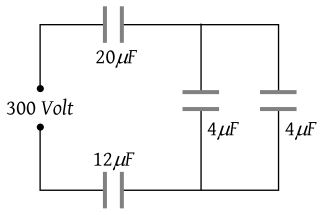(1) 1 μF

(2) $\frac{31}{120}$ μF

(3) 2 μF

(4) $\frac{120}{31}$μF

Concept Questions :-

Combination of capactiors
High Yielding Test Series + Question Bank - NEET 2020

Difficulty Level:

In the following circuit, the resultant capacitance between A and B is -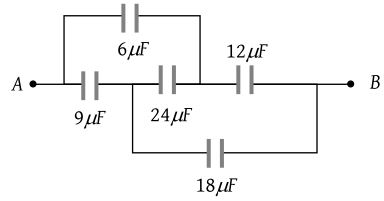(1) $\frac{32}{11}\mu F$

(2) $\frac{11}{32}\mu F$

(3) $\frac{23}{32}\mu F$

(4)

Difficulty Level:

A capacitor is charged by a battery. The battery is removed and another identical uncharged capacitor is connected in parallel. The total electrostatic energy of the resulting system

1. increases by a factor of 4

2.decreases by a factor of 2

3. remain the same

4. increases by a factor of 2

Concept Questions :-

Combination of capactiors
High Yielding Test Series + Question Bank - NEET 2020

Difficulty Level:

The diagrams below show regions of equipotentials.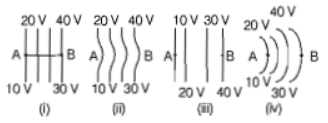A positive charge is moved from A to B in each diagram.

(a) Maximum work is requried to move q in figure(iii)

(b) In all the four cases,the work done is the same

(c) Minimum work is requried to move q in the figure(i)

(d) Maximum work is required to move q in figure(ii)

Concept Questions :-

Equipotential surfaces
High Yielding Test Series + Question Bank - NEET 2020

Difficulty Level:

A parallel-plate capacitor of area A, plate separation d, and capacitance C is filled with four dielectric materials having dielectric constants and ${k}_{4}$ as shown in the figure below. If a single dielectric material is to be used to have the same capacitance C in this capacitor, then its dielectric constant k is given by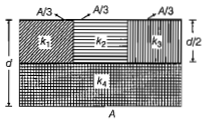(a) $k={k}_{1}+{k}_{2}+{k}_{3}+3{k}_{4}$

(b) $k=\frac{2}{3}\left({k}_{1}+{k}_{2}+{k}_{3}\right)+2{k}_{4}$

(c) $\frac{2}{k}=\frac{3}{{k}_{1}+{k}_{2}+{k}_{3}}+\frac{1}{{k}_{4}}$

(d) $\frac{1}{k}=\frac{1}{{k}_{1}}+\frac{1}{{k}_{2}}+\frac{1}{{k}_{3}}+\frac{3}{2{k}_{4}}$

Concept Questions :-

Dielectrics
High Yielding Test Series + Question Bank - NEET 2020

Difficulty Level:

A capacitor of 2$\mu F$ is charged as shown in the figure. When the switch S is turned to position 2, the percentage of its stored energy dissipated is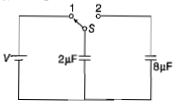1. 20%               2. 75%

3. 80%               4. 0%

Concept Questions :-

Energy stored in capacitor
High Yielding Test Series + Question Bank - NEET 2020

Difficulty Level:

A parallel plate air capacitor of capacitance C is connected, to a cell of emf V and then disconnected from it. A dielectric slab of dielectric constant K, which can just fill the air gap of the capacitor, is now inserted in it. Which of the following is incorrect?

(a) The potential difference between the plates decreases K times

(b) The energy stored in the capacitor decreases K times

(c) The change in energy stored is $\frac{1}{2}$CV2$\left(\frac{1}{K-1}\right)$

(d) The charge on the capacitor is not conserved

Concept Questions :-

Energy stored in capacitor
High Yielding Test Series + Question Bank - NEET 2020

Difficulty Level:

If potential (in volts) in a region is expressed as V(x,y,z)=6xy-y+2yz, the electric field (in N/C) at point (1,1,0) is

(a)-(3$\stackrel{^}{i}$+5$\stackrel{^}{j}$+3$\stackrel{^}{\mathrm{k}}$)
(b)-(6$\stackrel{^}{i}$+5$\stackrel{^}{j}$+2$\stackrel{^}{\mathrm{k}}$)
(c)-(2$\stackrel{^}{i}$+3$\stackrel{^}{j}$+$\stackrel{^}{\mathrm{k}}$)
(d)-(6$\stackrel{^}{i}$+9$\stackrel{^}{j}$+$\stackrel{^}{\mathrm{k}}$

Concept Questions :-

Relation between field and potential
High Yielding Test Series + Question Bank - NEET 2020

Difficulty Level:

A parallel plate air capacitor has capacity C, distance of separation between plates is d and potential difference V is applied between the plates. Force of attraction between the plates of the parallel plate air capacitor is

(a)C2V2/2d

(b)CV2/2d

(c)CV2/d

(d)C2V2/2d2

High Yielding Test Series + Question Bank - NEET 2020

Difficulty Level:

Two thin dielectric slabs of dielectric constants K1&K2 (${K}_{1}<{K}_{2}$) are inserted between plates of a parallel capacitor, as shown in the figure. The variation of electric field E between the plates with distance d as measured from plate P is correctly shown by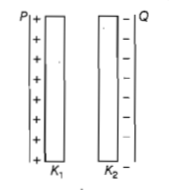1.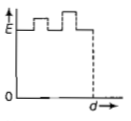2.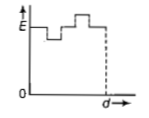3.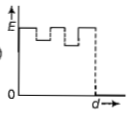4.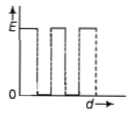Concept Questions :-

Dielectrics